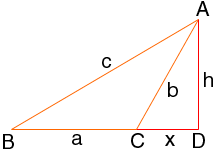Name: Bryant Who is asking: Other Level: Secondary Question: The question that I am pondering is that I need to derive the law of cosines for a case in which angle C is an obtuse angle. c 2 = a 2 + b 2 - 2ab cos C cos(180 degrees - C) = -cos C Hi, In the diagram below of a triangle with angle C obtuse, I extended the line segment BC drew a perpendicular from A to meet this extended line at D. Let x be the length of CD and h the length of AD.Triangle ABD is a right triangle and hence c 2 = (a + x) 2 + h 2 = a 2 + 2ax + x 2 + h 2 Triangle ACD is also a right triangle and thus b 2 = x 2 + h 2 Hence c 2 = a 2 + b 2 +2ax Can you complete the proof? Penny Go to Math Central